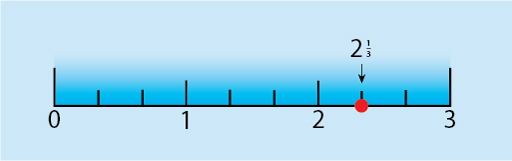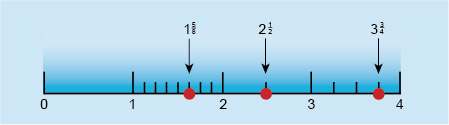Succeed with maths – Part 1

Start this free course now. Just create an account and sign in. Enrol and complete the course for a free statement of participation or digital badge if available.

4 Mixed numbers

In most of the examples considered so far, you have been using fractions to describe part of a whole. The result is a positive number whose value is less than 1. Fractions in which the numerator (top number) is less than the denominator (bottom number), such as and , are known as proper fractions.

A mixed number consists of a whole number and a proper fraction, for example . This means two and one third.

Mixed numbers can be represented on a number line, as shown here, by dividing each unit interval into parts. For example, to mark on the number line, the interval from 2 to 3 can be divided into thirds.

Then, can be marked at the point one-third of the way along the interval from 2 to 3.Figure 11 Number line showing 2 and one-third

You can get a better feel for this in the next activity.

Activity 5 Fractions on the number line

Timing: Allow approximately 10 minutes

Draw a number line from 0 to 5. Leave enough space between the numbers to be able to divide an interval into 8 parts. Now, plot the following fractions on the number line:

Comment

Which two whole numbers does lie between? Into how many parts do you want to divide the distance between these whole numbers if you have quarters?

You can determine the position of a number by dividing the length between the two appropriate whole numbers on the scale into 2, 4 or 8 equal parts (or pieces) as appropriate, and then plotting the fractions on it as shown below.Figure 12 A number line with answers

You can visualise these fractions again by using pizzas. For example, would be two whole pizzas plus one half pizza.

What if the numerator (the number on the top of the fraction) is more than the denominator? What kind of fraction is that? You’ll find the answer to this question in the next section.